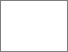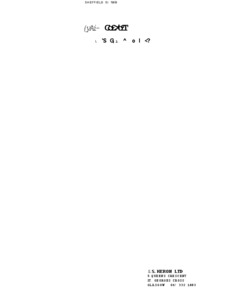# Design of discrete time controllers and estimators.

MOIR, Thomas J. (1982). Design of discrete time controllers and estimators. Doctoral, Sheffield Hallam University (United Kingdom)..Preview
PDF (Version of Record)
10697382.pdf - Accepted Version

## Abstract

This thesis considers optimal linear least-squares filtering smoothing prediction and regulation for discrete-time processes. A finite interval smoothing filter is derived in the z domain giving a transfer function solution. The resulting time-invariant smoother can be applied to problems where, a time varying solution using matrix Riccati equations would diverge if the process is modelled inaccurately. A self-tuning algorithm is given for the filtering and fixed lag smoothing problems as applied to square multi-variable ARMA processes when only the order of the process is assumed known. The dynamics of the process can also be slowly time varying. If the dynamics remain constant and unknown, it is shown how the self-tuning filter or smoother algorithm converges asymptotically to the optimal Wiener solutions. LQG self-tuning regulation is considered. The LQG algorithms rely on input-output data rather than from the conventional state-space approach employing the Kalman filter. An explicit algorithm is given which is similar to certain pole placement self-tuning regulators, requiring the solution of a diophantine equation. Following this, an implicit algorithm is shown to overcome the problem of solving a diophantine equation by estimating the regulator parameters directly using recursive least squares. The LQG algorithms are shown to be able to cope with processes which are non-minimum phase, open loop unstable and with an unknown time delay.

Item Type: Thesis (Doctoral) Thesis (Ph.D.)--Sheffield Hallam University (United Kingdom), 1982. Sheffield Hallam Doctoral Theses EPrints Services 10 Apr 2018 17:21 26 Apr 2021 12:08 https://shura.shu.ac.uk/id/eprint/20075View Item